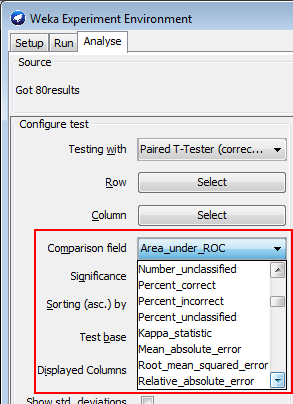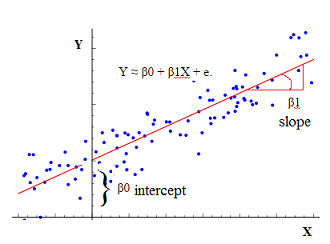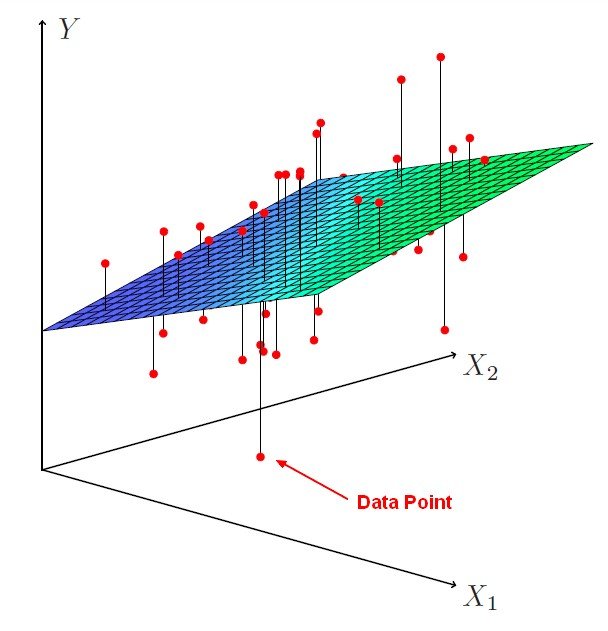# Statistics - R (Big R)

R (Big R) is a complex model with multiple correlation coefficient. R is an accuracy statistic for the overall model.

R is:

• the correlation between the predicted scores and the observed scores

R tells:

• how well the model are doing in predicting the observed scores.

For a simple regression, R (Big R) is just the correlation coefficient, little r squared but when we build bigger models with more and more predictors we will get a multiple correlation coefficient and particularly R squared.

Recommended PagesData Mining - (Parameters | Model) (Accuracy | Precision | Fit | Performance) Metrics

Accuracy is a evaluation metrics on how a model perform. rare event detection Hypothesis testing: t-statistic and p-value. The p value and t statistic measure how strong is the...Statistics - (Univariate|Simple|Basic) Linear Regression

A Simple Linear regression is a linear regression with only one predictor variable (X). Correlation demonstrates the relationship between two variables whereas a simple regression provides an equation...Statistics - Multiple Linear Regression

Multiple regression is a regression with multiple predictors. It extends the simple model. You can have many predictor as you want. The power of multiple regression (with multiple predictor) is to better...